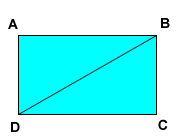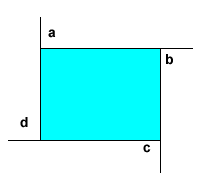# Interior Angles of a Polygon

### Quick Definitions

Let's go over a few key words so we're all on the same page. Remember that a polygon is a two-dimensional shape with sides drawn by straight lines (no curves) which together form a closed area. Each point on a polygon where two sides meet is called a vertex. At each vertex, there is an interior angle of the polygon. A square, for example, has four interior angles, each of 90 degrees. If the square represented your classroom, the interior angles are the four corners of the room.

### Sum of the interior angles

To extend that further, if the polygon has x sides, the sum, S, of the degree measures of these x interior sides is given by the formula S = (x - 2)(180).

For example, a triangle has 3 angles which add up to 180 degrees. A square has 4 angles which add up to 360 degrees. For every additional side you add, you have to add another 180 degrees to the total sum.

Let's talk about a diagonal for a minute. What is a diagonal anyway? A diagonal is a line segment connecting two nonconsecutive vertices of the polygon. It's all the lines between points in a polygon if you don't count those that are also sides of the polygon. In the picture below, BD is a diagonal. As you can see, line segment BD divides quadrilateral ABCD into two triangles. The sum of the angles in those triangles (180+180=360) is the same as the sum of all the angle measures of the rectangle (360).## Example 1

Quadrilateral ABCD has, of course, four angles. Those four angles are in the ratio 2:3:3:4. Find the degree measure of the biggest angle of quadrilateral ABCD.

### What do we know?

We have four unknown angles, but information about their relationship to each other. Since we know the sum of all four angles must be 360 degrees, we just need an expression which adds our four unknown angles and sets them equal to 360. Because they are in a ratio, they must have some common factor that we need to find, called x.

### Steps:

1. Add the terms 2x + 3x + 3x + 4x
2. Equate the sum of the terms to 360
3. Solve for x
4. Determine the angle measures in degrees.

### Solve

2x + 3x + 3x + 4x = 360
12x = 360
x = 360/12
x = 30

Even though we know x = 30 we aren't done yet. We multiply 30 times 4 to find the biggest angle. Since 30 times 4 = 120, the biggest angle is 120 degrees. Likewise, the other angles are 3*30=90, 3*30=90, and 2*30 = 60.

### Regular Polygons

A regular polygon is equiangular. All of its angles have the same measure. It is also equilateral. All of its sides have the same length. A square is a regular polygon, and while a square is a type of rectangle, rectangles which are not squares would not be regular polygons.

## Example 2

Find the sum of the degree measures of the angles of a hexagon. Assuming the hexagon is regular, find the degree measure of each interior angle.

### What do we know?

We can use the formula S = (x - 2)(180) to sum the degree measure of any polygon.

A hexagon has 6 sides, so x=6.

### Solve

Let x = 6 in the formula and simplify:

S = (6 - 2)(180)
S = 4(180)
S = 720

A regular polygon is equiangular, which means all angles are the same measure. In the case of a regular hexagon, the sum of 720 degrees would be distributed evenly among the six sides.

So, 720/6 = 120. There are six angles in a regular hexagon, each measuring 120 degrees.

## Example 3

If the sum of the angles of a polygon is 3600 degrees, find the number of sides of the polygon.

### Reversing the formula

Again, we can use the formula S = (x - 2)(180), but this time we're solving for x instead of S. No big deal!

### Solve

In this problem, let S = 3600 and solve for x.

3600 = (x - 2)(180)
3600 = 180x - 360
3600 + 360 = 180x
3960 = 180x
3960/180 = x
22 = x

A polygon with 22 sides has 22 angles whose sum is 3600 degrees.

### Exterior Angles of a Polygon

At each vertex of a polygon, an exterior angle may be formed by extending one side of the polygon so that the interior and exterior angles at that vertex are supplementary (add up to 180). In the picture below, angles a, b c and d are exterior and the sum of their degree measures is 360.If a regular polygon has x sides, then the degree measure of each exterior angle is 360 divided by x.

Let's look at two sample questions.

## Example 4

Find the degree measure of each interior and exterior angle of a regular hexagon.

Remember the formula for the sum of the interior angles is S=(x-2)*180. A hexagon has 6 sides. Since x = 6, the sum S can be found by using S = (x - 2)(180)

S = (10 -6)(180)
S = 4(180)
S = 720

There are six angles in a hexagon, and in a regular hexagon they are all equal. Each is 720/6, or 120 degrees. We now know that interior and exterior angles are supplementary (add up to 180) at each vertex, so the measure of each exterior angle is 180 - 120 = 60.

## Example 5

If the measure of each interior angle of a regular polygon is 150, find the number of sides of the polygon.

Previously we identified the number of sides in a polygon by taking the sum of the angles and using the S=(x-2)*180 formula to solve. But, this time we only know the measure of each interior angle. We'd have to multiply by the number of angles to find the sum... but the whole problem is that we don't know the number of sides yet OR the sum!

But, because the measure of each interior angle is 150, we also know the measure of an exterior angle drawn at any vertex in terms of this polygon is 180 - 150 = 30. That's because they form supplementary pairs (interior+exterior=180).

Before example 4, we learned that we can also calculator the measure of an exterior angle in a regular polygon as 360/x, where x is the number of sides. Now we have a way to find the answer!

30 = 360/x
30x = 360
x = 360/30
x = 12

Our polygon with 150 degree interior angles (and 30 degrees exterior angles) has 12 sides.

By the way, a geometric figure with 12 sides is called a dodecagon.

A lesson provided by Mr. Feliz